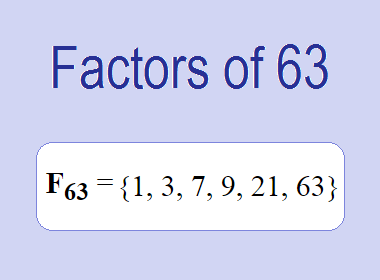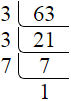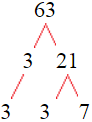# Factors of 63The factors of 63 are 1, 3, 7, 9, 21, and 63 i.e. F63 = {1, 3, 7, 9, 21, 63}. The factors of 63 are those numbers that can divide 63 without leaving a remainder.

We can check if these numbers are factors of 63 by dividing 63 by each of them. If the result is a whole number, then the number is a factor of 63. Let's do this for each of the numbers listed above:

·        1 is a factor of 63 because 63 divided by 1 is 63.

·        3 is a factor of 63 because 63 divided by 3 is 21.

·        7 is a factor of 63 because 63 divided by 7 is 9.

·        9 is a factor of 63 because 63 divided by 9 is 7.

·        21 is a factor of 63 because 63 divided by 21 is 3.

·        63 is a factor of 63 because 63 divided by 63 is 1.

## How to Find Factors of 63?

1 and the number itself are the factors of every number. So, 1 and 63 are two factors of 63. To find the other factors of 63, we can start by dividing 63 by the numbers between 1 and 63. If we divide 63 by 2, we get a remainder of 1. Therefore, 2 is not a factor of 63. If we divide 63 by 3, we get a remainder of 0. Therefore, 3 is a factor of 63.

Next, we can check if 4 is a factor of 63. If we divide 63 by 4, we get a remainder of 3. Therefore, 4 is not a factor of 63. We can continue this process for all the possible factors of 63.

Through this process, we can find that the factors of 63 are 1, 3, 7, 9, 21, and 63. These are the only numbers that can divide 63 without leaving a remainder.

********************

********************

## Properties of the Factors of 63

The factors of 63 have some interesting properties. One of the properties is that the sum of the factors of 63 is equal to 104. We can see this by adding all the factors of 63 together:

1 + 3 + 7 + 9 + 21 + 63 = 104

Another property of the factors of 63 is that they are all odd numbers. This is because 63 is an odd number, and any even number cannot divide an odd number without leaving a remainder.

Another property of the factors of 63 is that there are only two prime factor of 63 which are 3 and 7.

## Applications of the Factors of 63

The factors of 63 have several applications in mathematics. One of the applications is in finding the highest common factor (HCF) of two or more numbers. The HCF is the largest factor that two or more numbers have in common. For example, to find the HCF of 63 and 45, we need to find the factors of both numbers and identify the largest factor they have in common. The factors of 63 are 1, 3, 7, 9, 21, and 63. The factors of 45 are 1, 3, 5, 9, 15, and 45. The largest factor that they have in common is 9. Therefore, the HCF of 63 and 45 is 9.

Another application of the factors of 63 is in prime factorization. Prime factorization is the process of expressing a number as the product of its prime factors. The prime factors of 63 are 3 and 7. We can express 63 as:

63 = 3 × 3 × 7

We can do prime factorization by division and factor tree method also. Here is the prime factorization of 63 by division method,63 = 3 × 3 × 7

Here is the prime factorization of 63 by the factor tree method,63 = 3 × 3 × 7

## Conclusion

The factors of 63 are the numbers that can divide 63 without leaving a remainder. The factors of 63 are 1, 3, 7, 9, 21, and 63. The factors of 63 have some interesting properties, such as being odd numbers and having a sum of 104. The factors of 63 have several applications in mathematics, such as finding the highest common factor and prime factorization.